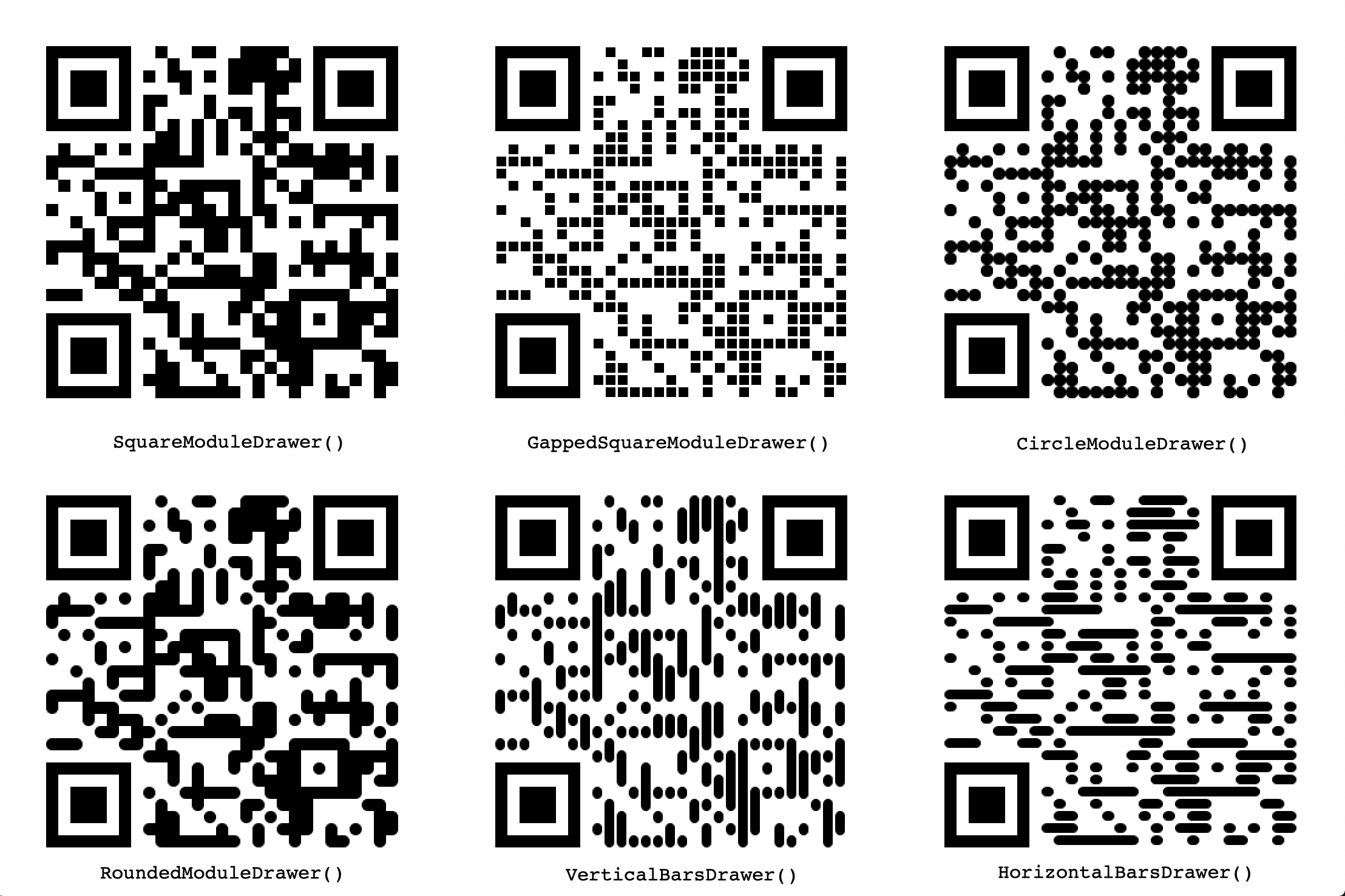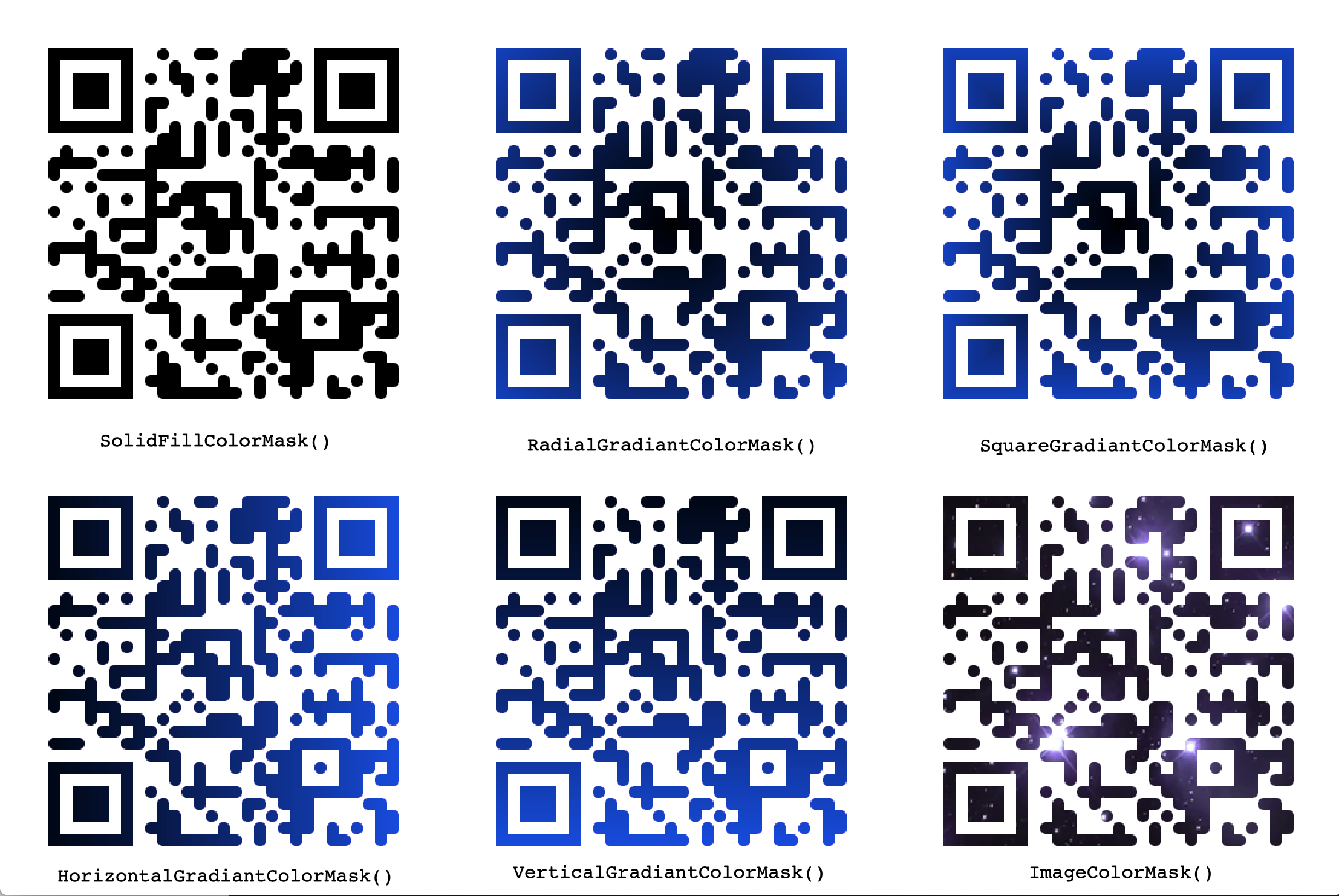﻿ 纯python二维码生成器 (google翻译) Pure python QR Code generator - 兰雅sRGB个人笔记

C, C++, Python Programming, Source Code, Video

# 纯python二维码生成器 Pure python QR Code generator

``````pip install qrcode

``````

# 用法

``````qr "Some text" > test.png
``````

``````import qrcode
img = qrcode.make('Some data here')
type(img)  # qrcode.image.pil.PilImage
img.save("some_file.png")
``````

## 高级用法

``````import qrcode
qr = qrcode.QRCode(
version=1,
error_correction=qrcode.constants.ERROR_CORRECT_L,
box_size=10,
border=4,
)
qr.make(fit=True)

img = qr.make_image(fill_color="black", back_color="white")

``````

`version` 参数是一个 1 到 40 之间的整数，用于控制 QR 码的大小（最小的版本 1 是一个 21x21 矩阵）。

`fill_color``back_color` 可以在使用默认图像工厂时更改 QR 的背景和绘画颜色。这两个参数都接受 RGB 颜色元组。

``````img = qr.make_image(back_color=(255, 195, 235), fill_color=(55, 95, 35))
``````

`error_correction` 参数控制用于二维码的纠错。 `qrcode` 包中提供了以下四个常量：

`ERROR_CORRECT_L`

``大约可以纠正 7% 或更少的错误。``

`ERROR_CORRECT_M` (default)

``大约 15% 或更少的错误可以被纠正。``

`ERROR_CORRECT_Q`

``大约 25% 或更少的错误可以被纠正。``

`ERROR_CORRECT_H`.

``````大约可以纠正 30% 或更少的错误。
``````

`box_size`参数控制二维码的每个 `box` 有多少像素。

`border`参数控制边框应该有多少个框厚（默认为 4，这是根据规范的最小值）。

# 其他图像工厂

SVG

``````qr --factory=svg-path "Some text" > test.svg
qr --factory=svg "Some text" > test.svg
qr --factory=svg-fragment "Some text" > test.svg
``````

``````import qrcode
import qrcode.image.svg

if method == 'basic':
# Simple factory, just a set of rects.
factory = qrcode.image.svg.SvgImage
elif method == 'fragment':
# Fragment factory (also just a set of rects)
factory = qrcode.image.svg.SvgFragmentImage
else:
# Combined path factory, fixes white space that may occur when zooming
factory = qrcode.image.svg.SvgPathImage

img = qrcode.make('Some data here', image_factory=factory)
``````

``````qrcode.image.svg.SvgFillImage
qrcode.image.svg.SvgPathFillImage

``````

## Pure Python PNG

``````pip install -e git+git://github.com/ojii/pymaging.git#egg=pymaging
pip install -e git+git://github.com/ojii/pymaging-png.git#egg=pymaging-png
``````

``````qr --factory=pymaging "Some text" > test.png
``````

``````import qrcode
from qrcode.image.pure import PymagingImage
img = qrcode.make('Some data here', image_factory=PymagingImage)

``````

## 样式图像

``````import qrcode
from qrcode.image.styledpil import StyledPilImage
from qrcode.image.styles.moduledrawers import RoundedModuleDrawer

qr = qrcode.QRCode(error_correction=qrcode.constants.ERROR_CORRECT_L)

img_1 = qr.make_image(image_factory=StyledPilImage, module_drawer=RoundedModuleDrawer())
img_3 = qr.make_image(image_factory=StyledPilImage, embeded_image_path="/path/to/image.png")
``````# 例子

`print_ascii` 获取文本内容:

``````import io
import qrcode
qr = qrcode.QRCode()
f = io.StringIO()
qr.print_ascii(out=f)
f.seek(0)
``````

`add_data` 方法将数据附加到当前 QR 对象。要通过替换同一对象中以前的内容来添加新数据，请首先使用 `clear` 方法：

``````import qrcode
qr = qrcode.QRCode()
img = qr.make_image()
qr.clear()
other_img = qr.make_image()
``````

``````qr --ascii "Some data" > "test.txt"
cat test.txt
``````

``````# qr "Some data" > test.png
qr --output=test.png "Some data"
``````